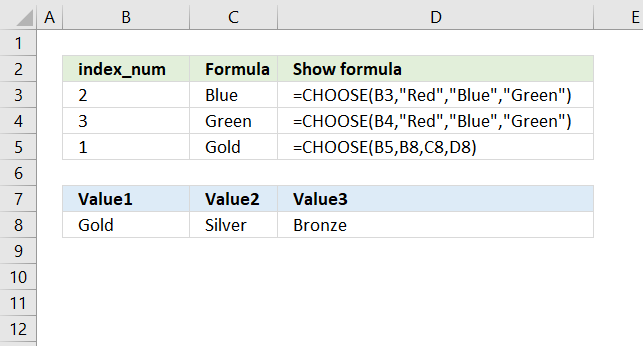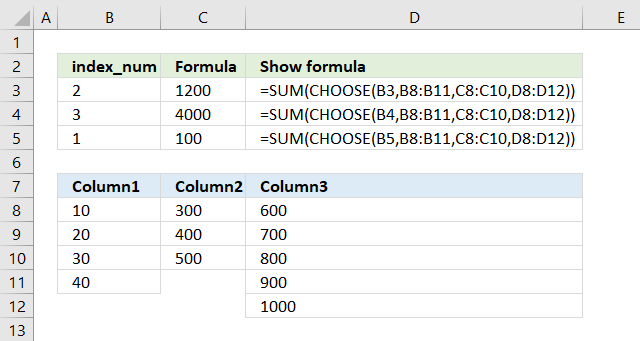Author: Oscar Cronquist Article last updated on March 29, 2018The CHOOSE function allows you to retrieve a value based on a number, cell range C3:C4 in the picture above shows you a formula that has values "hard-coded" into the formula.

Cell C5 has a formula that shows a value from row 8 using cell references.

Note, you can't use a cell range containing multiple values. You need to type each cell separated by a comma.

### Excel function syntax

CHOOSE(index_num, value1, [value2], ...)

### Arguments

Index_num - Determines which value is chosen. This argument is required, you can use a number between 1 and 254.

value1, value2, value3, ... - Up to 254 values Index_num can pick from. The first value is required the remaining values are optional.

### ExampleThe picture above displays a formula that allows you to select a cell range and then a SUM function adds all numbers in the selected cell range.

Formula in cell C3 selects cell range C8:C10 and adds the numbers 300 + 400 + 500 = 1200 is shown in cell C3.

### Tip!

Use function key F9 to quickly create hard-coded values from a specific cell range, then use the values in the CHOOSE function.

1. Double-click on an empty cell
2. Type = (equal sign)
3. Select a cell range with your mouse or type a cell range
4. Press F9 to convert values in cell range to "constants" or hard-coded values
5. Delete the curly brackets { }
6. Use the values in your CHOOSE function